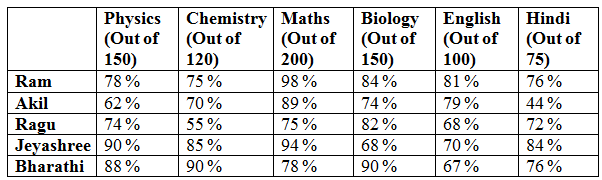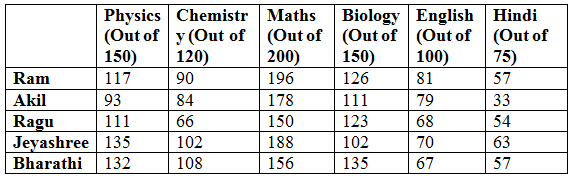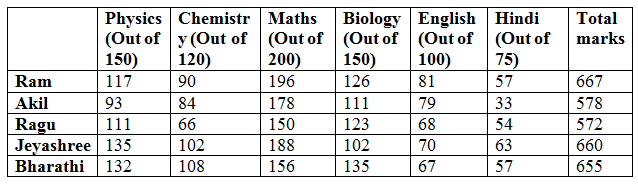# IBPS Clerk Prelims 2018 – Quantitative Aptitude Questions Day-49

Dear Readers, Bank Exam Race for the Year 2018 is already started, To enrich your preparation here we have providing new series of Practice Questions on Quantitative Aptitude – Section. Candidates those who are preparing for IBPS Clerk Prelims 2018 Exams can practice these questions daily and make your preparation effective.

[WpProQuiz 4551]

Directions (Q. 1 – 5): In each of these questions two equations (I) and (II) are given. You have to solve both the equations and give answer as,

a) If x > y

b) If x ≥ y

c) If x < y

d) If x ≤ y

e) If x = y or no relation can be established between x and y

1) I) x2 – x – 132 = 0

II) y2 + 16y – 57 = 0

2) I) 2x2 – 12x – 32 = 0

II) 3y2 – 15y + 18 = 0

3) I) 5x – y = -22

II) 3x + 2y = -21

4) I) 5x2 + 2x – 16 = 0

II) 4y2 + 8y – 28 = 0

5) I) (3375)1/3 x = √5625

II) y2 – 9y – 20 = 0

Directions (Q. 6 – 10): Study the following information carefully and answer the given questions.

The following table shows the percentage of marks taken by the students in different subjects in a certain examination.6) Find the total marks scored by Ram?

a) 667

b) 628

c) 553

d) 592

e) None of these

7) Total marks scored by Akil in the subjects of maths and biology is approximately what percentage of total marks scored by Bharathi in the same subjects?

a) 90 %

b) 120 %

c) 75 %

d) 100 %

e) 125 %

8) Find the ratio of total marks scored by all the students in maths to that of Chemistry?

a) 356 : 225

b) 485 : 127

c) 434 : 225

d) 398 : 127

e) None of these

9) Which of the following student gets highest total marks?

a) Akil

b) Jeyashree

c) Ragu

d) Bharathi

e) Ram

10) Total marks scored by Akil in all the given subject is approximately what percentage more/less than the total marks scored by Ragu in all the given subject?

a) 5 % more

b) 12 % less

c) 1 % less

d) 1 % more

e) 12 % more

Direction (1-5) :

I) x2 – x – 132 = 0

(x + 11) (x – 12) = 0

X = -11, 12

II) y2 + 16y – 57 = 0

(y + 19) (y – 3) = 0

Y = -19, 3

Can’t be determined

I) 2x2 – 12x – 32 = 0

2x2 + 4x – 16x – 32 = 0

2x(x + 2) – 16(x + 2) = 0

(2x – 16) (x + 2) = 0

X = 16/2, -2 = 8, -2

II) 3y2 – 15y + 18 = 0

3y2 – 9y – 6y + 18 = 0

3y (y – 3) – 6 (y – 3) = 0

(3y – 6) (y – 3) = 0

Y = 6/3, 3 = 2, 3

Can’t be determined

5x – y = -22 –> (1)

3x + 2y = -21 –> (2)

By solving the equation (1) and (2), we get,

X = -5, y = -3

X < y

I) 5x2 + 2x – 16 = 0

5x2 + 10x – 8x – 16 = 0

5x (x + 2) -8 (x + 2) = 0

(5x – 8) (x + 2) = 0

X = 8/5, -2 = 1.6, -2

II) 4y2 + 8y – 28 = 0

4y2 + 16y – 7y – 28 = 0

4y (y + 4) -7 (y + 4) = 0

(4y – 7) (y + 4) = 0

Y = 7/4, – 4 = 1.75, – 4

Can’t be determined

I) (3375)1/3 x = √5625

15x = 75

X = 75/15 = 5

II) y2 – 9y – 20 = 0

(y – 4) (y – 5) = 0

Y = 4, 5

X ≥ y

Direction (6-10) :Total marks scored by Ram = 117 + 90 + 196 + 126 + 81 + 57 = 667

Total marks scored by Akil in the subjects of maths and biology is,

= > 178 + 111 = 289

Total marks scored by Bharathi in the subjects of maths and biology is,

= > 156 + 135 = 291

Required % = (289/291)*100 = 99.31 = 100 %

Total marks scored by all the students in Maths,

= > 196 + 178 + 150 + 188 + 156 = 868

Total marks scored by all the students in Chemistry,

= > 90 + 84 + 66 + 102 + 108 = 450

Required ratio = 868 : 450 = 434 : 225Ram got a highest total marks.

Total marks scored by Akil in all the given subject is,

= > 93 + 84 + 178 + 111 + 79 + 33 = 578

The total marks scored by Ragu in all the given subject is,

= > 111 + 66 + 150 + 123 + 68 + 54= 572

Required % = [(578 – 572)/572]*100 = 1.048 % = 1 % more

Kindly watch this video to know about Memory Based Arithmetic Questions Asked in IBPS Clerk Pre 2018 Held on 8th Dec

Daily Practice Test Schedule | Good Luck

 Topic Daily Publishing Time Daily News Papers & Editorials 8.00 AM Current Affairs Quiz 9.00 AM Current Affairs Quiz (Hindi) 9.30 AM IBPS Clerk Prelims – Reasoning 10.00 AM IBPS Clerk Prelims – Reasoning (Hindi) 10.30 AM IBPS Clerk Prelims – Quantitative Aptitude 11.00 AM IBPS Clerk Prelims – Quantitative Aptitude (Hindi) 11.30 AM Vocabulary (Based on The Hindu) 12.00 PM IBPS Clerk Prelims – English Language 1.00 PM SSC Practice Questions (Reasoning/Quantitative aptitude) 2.00 PM IBPS Clerk – GK Questions 3.00 PM SSC Practice Questions (English/General Knowledge) 4.00 PM Daily Current Affairs Updates 5.00 PM Canara Bank PO Mains – Reasoning 6.00 PM Canara Bank PO Mains – Quantitative Aptitude 7.00 PM Canara Bank PO Mains – English Language 8.00 PM